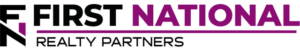# What is The Discount Rate in Commercial Real Estate?

### Key Takeaways

• The discount rate is a real estate metric that represents an investor’s required rate of return on an investment.  It is a key input in discounted cash flow analysis, which helps to determine a fair sales price for a property.
• An appropriate discount rate isn’t so much calculated as it is chosen based on a number of factors, including market conditions, the desired rate of return, and property specific risk.
• The discount rate is similar to another real estate performance metric known as the cap rate, but the key difference is that the cap rate is driven by the property’s market value while the discount rate is driven by an investor’s preferences.
• In a private equity transaction, the deal sponsor will choose an appropriate discount rate and use it to calculate a favorable purchase price for the property.

At its core, a commercial real estate investment is a trade of sorts.  When a real estate investor purchases a property, they trade a lump sum of money in the present for a periodic stream of income in the future.  Thus, one of the most important questions at the forefront of every investor’s mind is: what is an appropriate price to pay today for a future stream of income?  The technique used to answer this question seeks to discount future cash flows back to the present using a “discount rate.”

In this article, we will describe what the discount rate is, how it is calculated, why it matters in commercial real estate, and how it is used to determine the present value of a series of cash flows.  By the end, readers will have the information needed to use the discount rate technique as part of their own investment due diligence process.

At First National Realty Partners, we use the discount rate as part of the underwriting process in all of our deals.  Doing so helps us to identify the opportunities that have the highest chance of a profitable return for our investors.  To learn more about our current investment opportunities, click here.

## Discount Rate Definition

There are two ways to define the discount rate in real estate.  The first is to define the discount rate as the rate that is used to determine the present value of future cash flows.  The second is to think about the discount rate as the rate of return required by an investor to take the risk of purchasing a property.  Either way, the resulting discount rate percentage is used to determine the present value of a future stream of cash flows.

## Calculating the Discount Rate for Real Estate

It is important to separate the discount rate itself from the type of analysis in which it is used, which is called discounted cash flow analysis, or DCF for short.

In real estate, the discount rate isn’t as much calculated as it is chosen, and the choice is highly dependent upon the analyst’s evaluation of three factors:

1. desired rate of return,
2. perceived risk, and
3. market direction.

Additional detail on each of these factors is below.

### 1. Desired Rate of Return

The real estate discount rate is heavily influenced by the annual return that an investor wishes to achieve.  Of the three factors described above, desired rate of return may be the most subjective because it is different for each investor.  One real estate investor may have a higher average cost of capital so they need to achieve a higher return.  Another investor may have a lower cost of capital so they are willing to accept a lower return.  So, when choosing an appropriate discount rate, investors should evaluate their own return objectives and choose a rate that best suits them.

While this choice is subjective, it is also heavily influenced by perceived risk.

### 2. Perceived Property Risk

For real estate deals with higher risk, investors demand a higher return, so they would choose a higher discount rate, which results in a lower present value (lower purchase price).  Conversely, if real estate investors believe that a deal has less risk, they will choose a lower discount rate, which results in a higher present value/purchase price.

### 3. Real Estate Market Base & Direction

If a real estate market or sub-market is strong and growing quickly, an investor may have to choose a lower discount rate to account for the purchase competition.  Ultimately, this means that they will pay a higher price.  Conversely, if the market is stagnant, an investor may choose a higher discount rate, which means their proposed purchase price is lower.

When choosing an appropriate discount rate for a commercial property, the important point is that the choice of a discount rate is somewhat subjective.  There is not necessarily a right or wrong answer, just one that is supported by market data, investor requirements, and logic.

For context, the discount rate for most commercial real estate transactions falls between a general range of 5% and 12%.

## Using the Discount Rate for Property Valuation

The idea behind using the discount rate to determine property valuation is that a property’s value is equal to the discounted value (using the discount rate) of the future cash flows it produces.  Mathematically, the property valuation formula looks like this: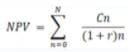While this formula looks intimidating, it doesn’t need to be.  It is rarely calculated manually.  Instead, the present value of the cash flows are calculated using the “NPV” function in a spreadsheet program like Excel or Google Sheets.  There are three variables needed to make the calculation:  the cash flows for each period, the discount rate, and the number of periods.

Cash flows are derived from the proforma, which is a financial model that projects an investment property’s income and operating expenses over a defined holding period.  The difference between income and operating expenses is known as net operating income (NOI), which is calculated on an annual basis.

The discount rate, as described above, is the rate at which the cash flows will be discounted, and it is chosen by the analyst as part of the DCF analysis.

Finally, the holding period is the number of years that the investor plans to hold the property.  This is also somewhat subjective, but a typical holding period for a commercial real estate property is five to ten years.

To illustrate how DCF analysis works, an example is helpful.

### DCF Analysis Example

Suppose that a real estate investor is considering the purchase of a property that produces cash flows of \$100,000, \$115,000, \$125,000, \$140,000, and \$150,000.  Also, assume that the investor has a required rate of return of 8%, which will be the discount rate.  So, the question is, at an 8% discount rate, what is this series of cash flows worth today?  The following table summarizes the answer:

 Year Cash Flow 1 \$100,000 2 \$115,000 3 \$125,000 4 \$140,000 5 \$150,000 NPV \$495,407.25

The net present value (NPV) of this series of property cash flows, at an 8% discount rate, is \$495,407.  But, this doesn’t necessarily mean that this is the price that should be offered for this property.  Instead, it is one input into the decision and should be considered along with a variety of other factors, including the cap rate.

## Discount Rate vs. Cap Rate

The discount rate is sometimes compared to another commercial real estate metric known as the capitalization rate, or cap rate for short.

The cap rate describes the relationship between a real estate asset’s Net Operating Income and its market value.  Although both metrics provide an indication of a property’s annual rate of return, there is one key difference between the cap rate and discount rate.

The cap rate is market driven because value is a key component in the calculation.  The discount rate is more subjective because it is an investor’s required rate of return.  However, both cap rate and discount rate can be used to estimate the potential purchase price of a real estate property.  In fact, these metrics are often used together.

## Discount Rate and Private Equity Real Estate

For individual real estate investors, one of the major benefits of working with a private equity firm is that the firm does all of the upfront work of analyzing a property to see if it has the potential to be a good deal.  As part of this analysis, the firm will estimate income and expenses, create a proforma, forecast cash flows, and choose an appropriate discount rate for which to perform a full discounted cash flow analysis. The result of this DCF analysis provides an indication of the potential return and a reasonable value of the property.  But, this isn’t all private equity firms do.

The best firms also calculate a series of return metrics, including the internal rate of return (IRR), gross rent multiplier, equity multiple, and cash on cash return.  By doing this sort of fundamental analysis, private equity firms can add significant value to a real estate investment by identifying the deals with the potential for the highest returns and presenting those ones to their investors.

## Summary & Conclusions

• The discount rate is a commercial real estate metric that represents an investor’s required rate of return on an investment.  Discount rate is a key input in discounted cash flow (DCF) analysis, which helps to determine a fair sales price for a property.
• An appropriate discount rate isn’t so much calculated as it is chosen based on a number of factors, including market conditions, the desired rate of return, and property specific risk.
• The discount rate is similar to cap rate, another performance metric, but the key difference is that the cap rate is driven by the property’s market value while the discount rate is driven by an investor’s preferences.
• In a private equity transaction, the deal sponsor will choose an appropriate discount rate and use it to calculate a favorable purchase price for the property.

## Interested In Learning More?

First National Realty Partners is one of the country’s leading private equity commercial real estate investment firms. With an intentional focus on finding world-class, multi-tenanted assets well below intrinsic value, we seek to create superior long-term, risk-adjusted returns for our investors while creating strong economic assets for the communities we invest in.

Get instant access to all of our current and past commercial real estate deals.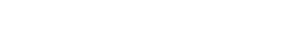## Subscribe Now

Get the latest news on real estate

Operations and Management

### October 9, 2023

FNRP Spotlight Series

1031 Exchange

## Get More From FNRP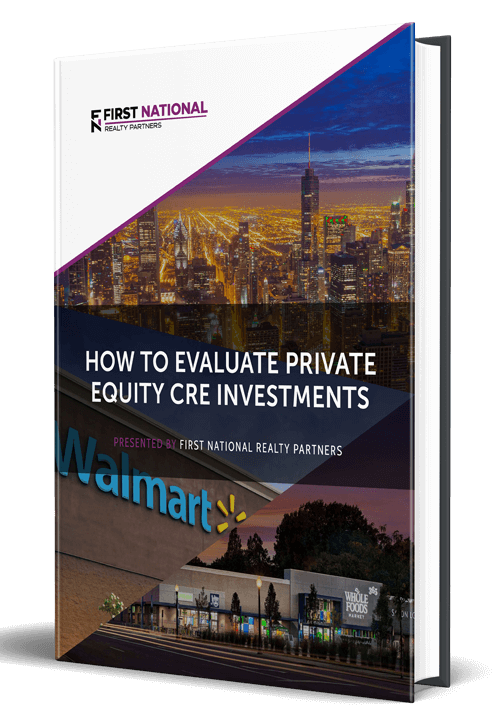## How to Evaluate Private Equity CRE Investments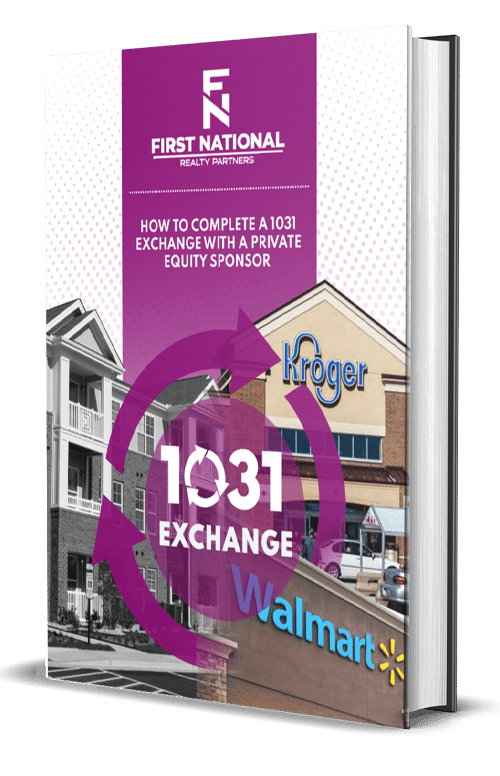## How to Complete a 1031 Exchange with a Private Equity Sponsor